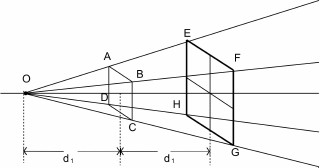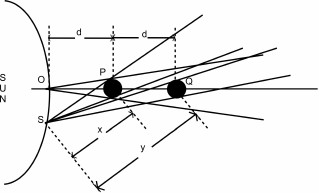Gravity from a new angle

# Inverse Square Law of Gravity

In Newtonian principle, force of gravity was proposed as inversely proportional to the square of the distance between the objects. If we double the distance between the objects, the strength of gravity will diminish to a one fourth of the original force between them.

A common example we find for the derivation of this principle is howFigure 1: Inverse Square Law

the intensity of light is diminished with the increase in distance between the light emitting point and an object with an area as shown in Fig 1. O is the light emitting point and ABCD is a square object.

The point O and the ABCD are separated by a distance of d1. If the square was moved away from O for a distance equal to d1, the luminosity of O on the square at new location will decrease to one fourth of the initial.

Gravity was also assumed to work in similar to the luminosity of a light source. In the previous example, O is a light emitting object and the square is a non-luminous object. Both are dissimilar objects by nature, means one emits the light and the other doesn't. In the case of gravity, both are mutually attracting objects. If the sun exerts gravity on the earth then the earth also exerts gravity on the sun. If we compare the gravity of an object with a light emitting from an object then both the sun and the earth should be treated as light emitting sources when calculating the gravity between them.

Another assumption made when deriving the inverse square law for gravity, apart from assuming that gravity is light, is that the light source was treated as a point. In reality, wherever we study the gravity between objects, none of them were of point mass objects. Consider the example of light reaching the Mercury from the sun as shown in Fig 2.

O and S are two different points on the surface of the sun and the d is the distance between the sun and the Mercury from point O. The distance between the sun and the Mercury from point S is x. If we double the distance of the Mercury from the sun from point O, the light reaching the Mercury from the sun wouldn't be 1/4th of the original, it will be slightly more than a quarter because the sun is emitting the lightFigure 2: Relevance of Inverse square law for the distance between Sun and Mercury.

in a wide angle instead of as a point. Even the new position of the Mercury wouldn't be exactly double from all other points on the sun. When the new position of the Mercury viewed from point S, the new distance y is not double the distance of the earlier distance of x. Therefore more light reaches the Mercury than 1/4th of the original.

As seen earlier in the chapter on mass, an object and its point mass counterpart are not same. When a bigger object turns to a point mass object, the new point mass object weighs more. According to the present definition of mass, the new point mass object gains more mass. Therefore, any principle derived assuming these two objects are same is invalid.

Gravity is not a thing that emanates all around an object like the light emanating from a light source. Light source emanates the light without any external influence. Gravity is a mutual entity. It will be felt on an object only when there is another object in its vicinity. If there is only one object, there wouldn't be any effect of gravity around it.

We see the waves in the ocean generated by the gravity between the earth and the moon travelling all around the earth due to the rotation of the earth and the revolution of the moon around the earth. If the earth and the moon remains stationary at a distance then the waves in the ocean always point towards the direction of the moon.

Another aspect which inverse square law ignored is the differential force of an object like planet Jupiter. Bigger objects will have both resultant and differential forces. A point size object will have only the resultant force. The effect of differential force is missing in the point mass object.

Gravity doesn't act like the light and the objects gravitationally interacting are not point mass objects. Therefore the inverse square law principle, which was derived based on the characteristics of the light emanating from a point source, wouldn't be valid for the gravity.

Date posted: October 1, 2009

Copyright 2009 Karunakar Marasakatla